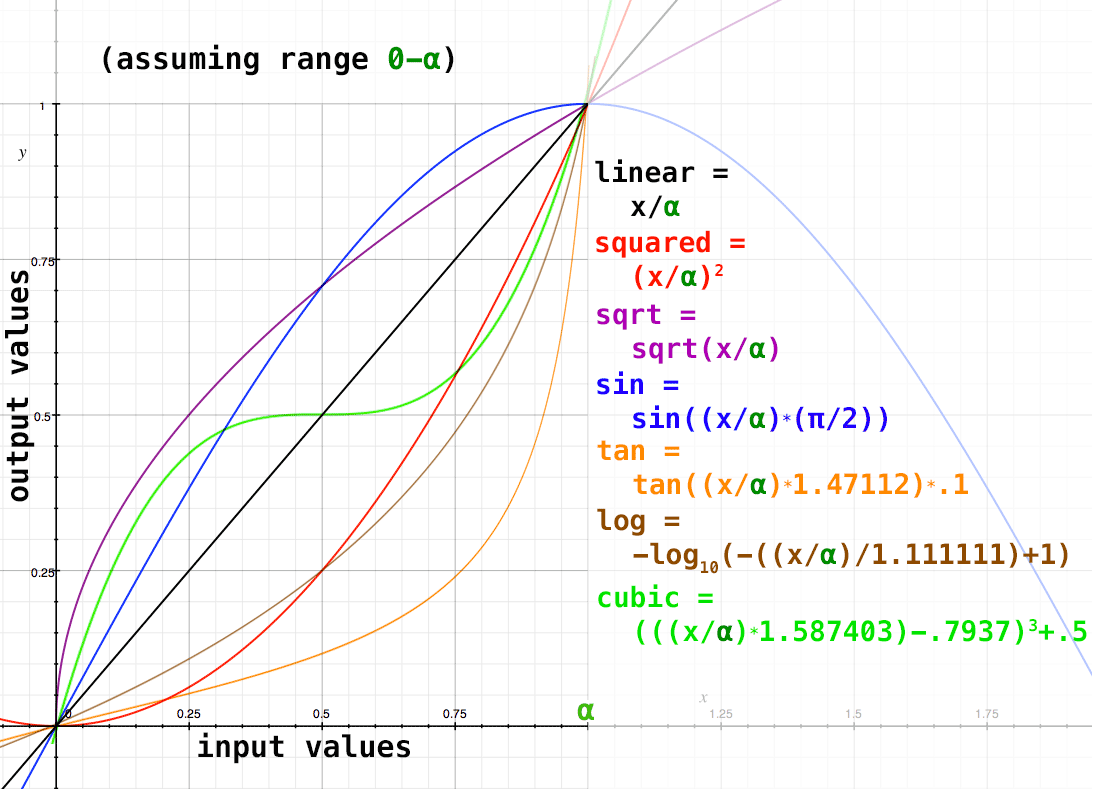Takes an input range of numbers (either fixed or on point attributes) and converts them to another range using equations to achieve different curves.## Parameters

 valueToSolve The value of x at which to solve the equation. Use Attribute If checked, will solve for the value listed in the attribute "solveAttrib". solveAttrib The point attribute containing the value to solve for.

### Main

 Output Attribute Point attribute in which to put the result. curveType Which curve to use to compute the result. See the above graph for an illustration of the options; the x axis is the inputs, and the y axis is the outputs. invert Check to invert (1-x) the outputs. Inverts across the x-axis while still outputting positive numbers.

### Input

 minInputValue The low-range of the input values. Use Attribute Whether to use an attribute for the low-range input, or a single number. minInputAttrib If "Use Attribute" is checked, it will read the point attribute listed here. maxInputValue The top-range of the input values. Use Attribute Whether to use an attribute for the top-range input, or a single number. maxInputAttrib If "Use Attribute" is checked, it will read the point attribute listed here.

### Output

 minOutputValue The low-range of the output values. Use Attribute Whether to use an attribute for the low-range output, or a single number. minOutputAttrib If "Use Attribute" is checked, it will read the point attribute listed here. maxOutputValue The top-range of the output values. Use Attribute Whether to use an attribute for the top-range output, or a single number. maxOutputAttrib If "Use Attribute" is checked, it will read the point attribute listed here.